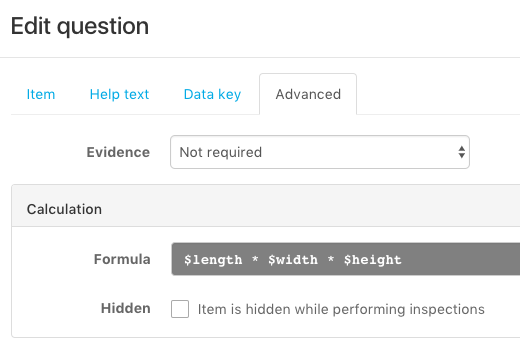Help Center

# Create a template: Formulas

For a numeric question, a formula can be used to calculate the answer based on other answers.

One could for example calculate the volume based on height, width & length using the formula \$length * \$width * \$height.

Note: The question values (length, width, height) are retrieved using their data keys. So data values should be enabled for both your organization (contact Support if needed) and the template itself.

### Hidden questions

When preferred the calculated question can be hidden so that the user won't see the output while performing the inspection.### A few things to note here:

• Variables that refer to an answer only work with questions that have a data key set and they must start with a \$, e.g.: \$length
• Formulas only result in an answer when all given variables have a value. In the example above, if either length, width or height was not answered, the result of the formula will simply be empty. In some cases you want to turn this around and make sure that all variables are interpreted as 0, to do so you can prefix the formula with a '@' so that the formula would look like:
@ \$length * \$width * \$height

### Expressions:

Besides usual mathematical expressions such as '\$a + \$b' or '(\$a + \$b + \$c) / \$d' you may also use additional helper expressions:

• \$prefix[] refer to a list of values. If you have 5 questions with data keys item_1, item_2, item_3, item_4 and item_5 with values 1, 2, 3, 4 and 5, the expression \$item[] will result in a list of all answers given to those questions.
NB: [] = square bracket open and square bracket closed!
• round( ... ), round a number to the nearest whole number
round(3.14159)3
• sum( ... ), sum a list of items
sum(\$item[])15 (sum of 1 + 2 + 3 + 4 + 5)
• count( ... ), count a list of items when answered
Suppose there is also a question with key item_6 which hasn't been answered yet, count(\$item[]) will result in 5